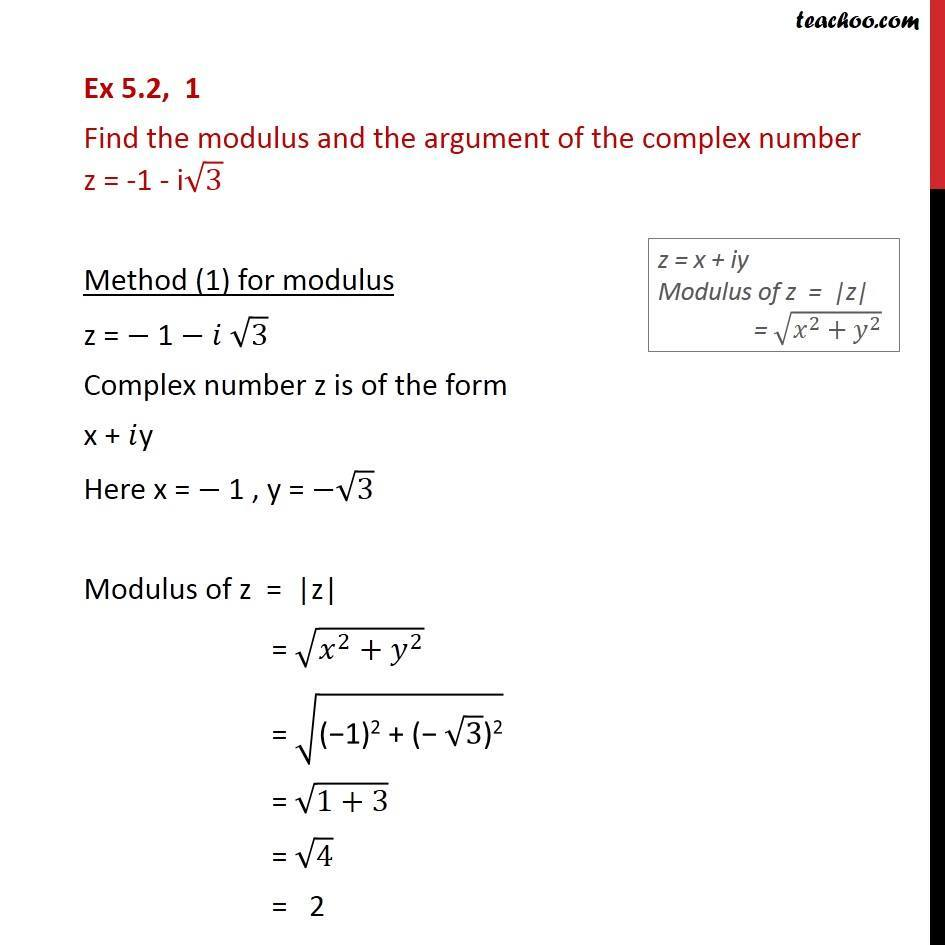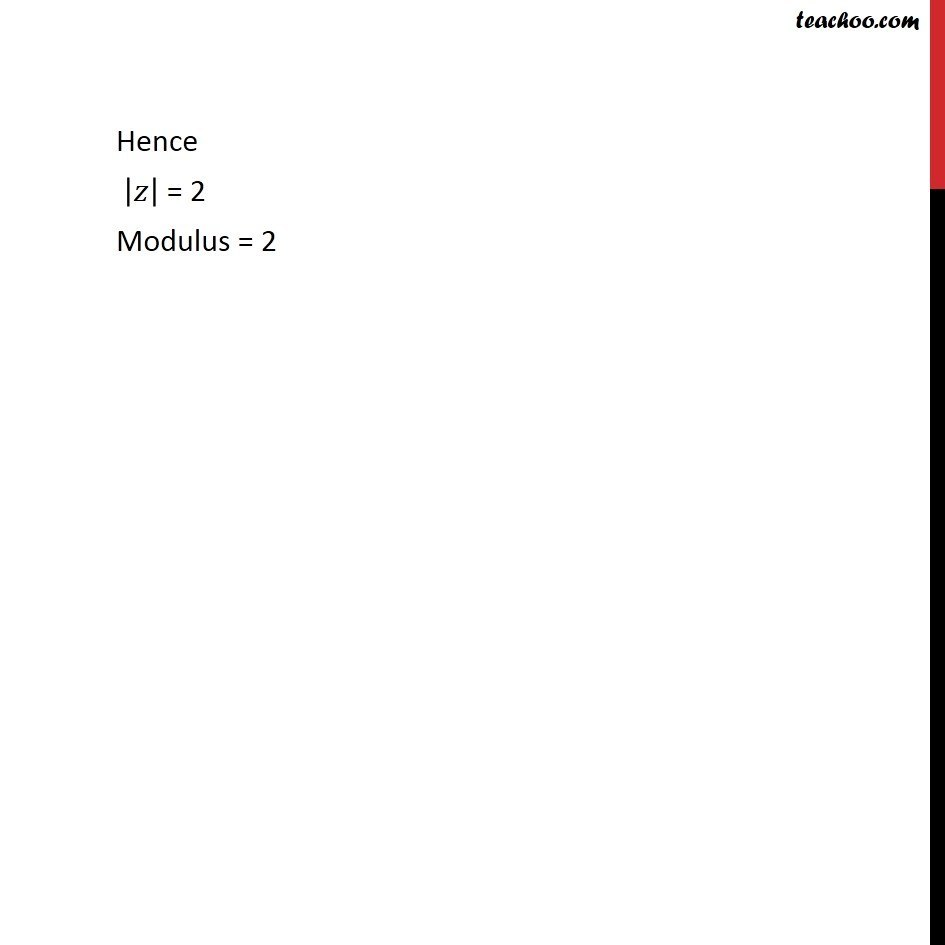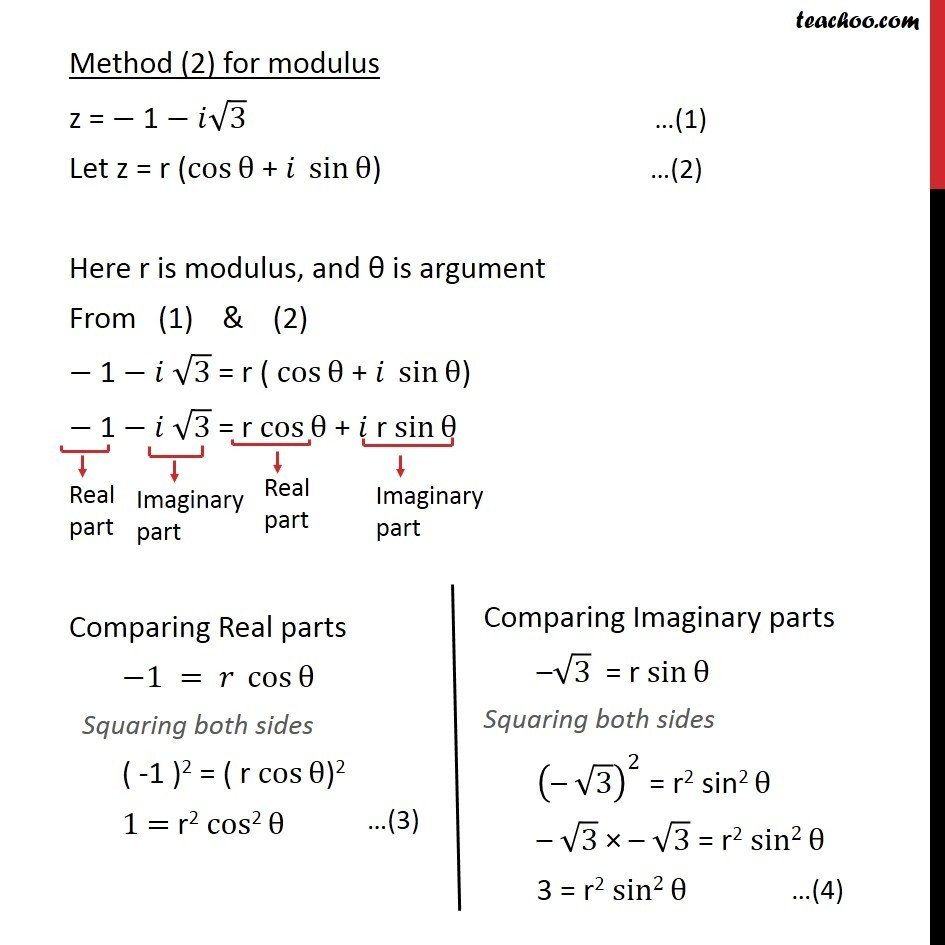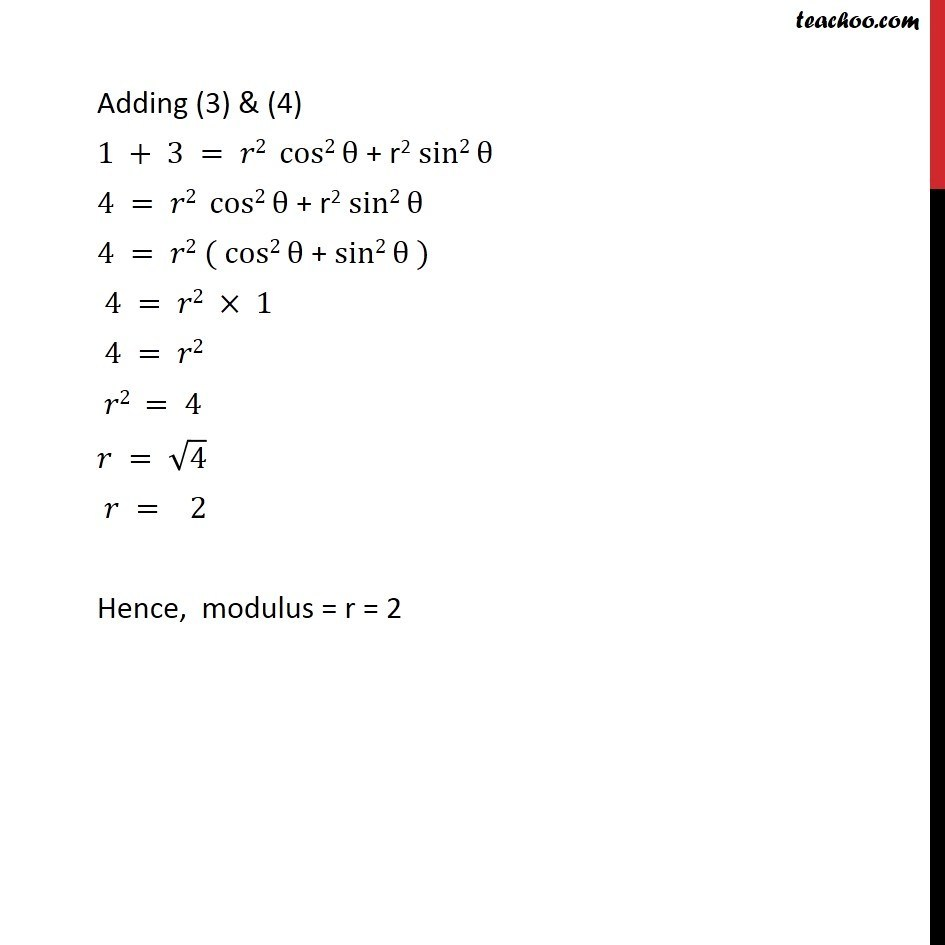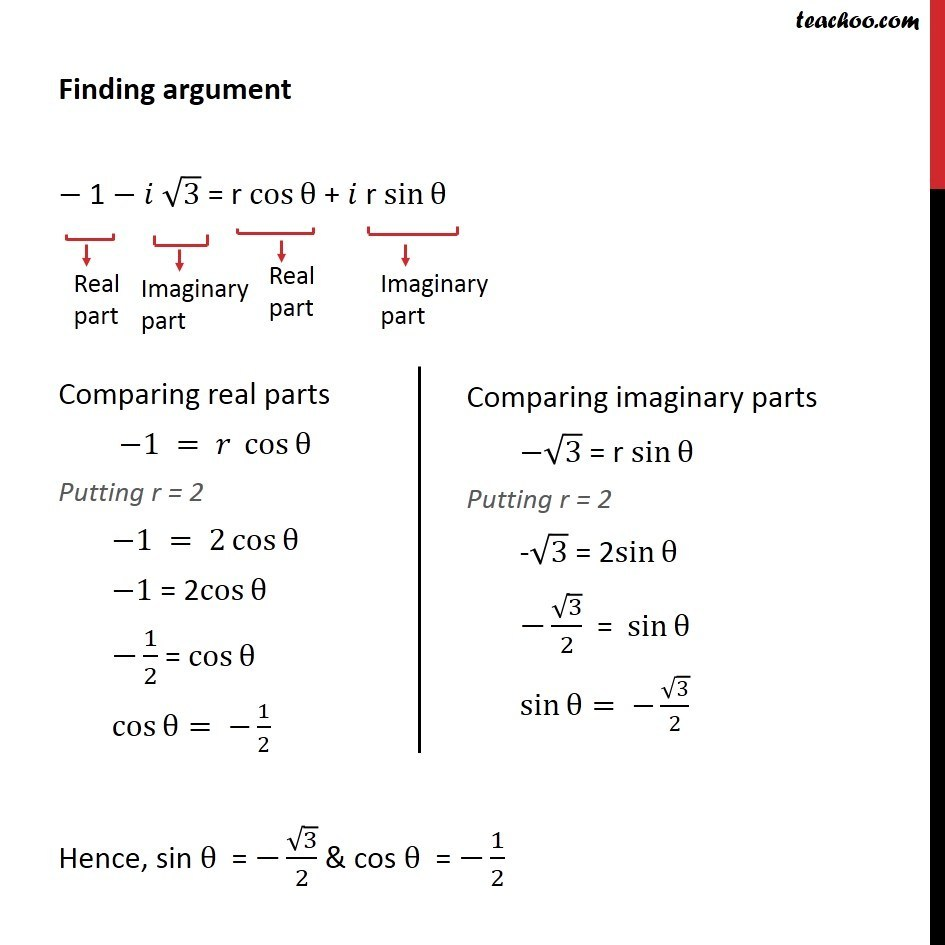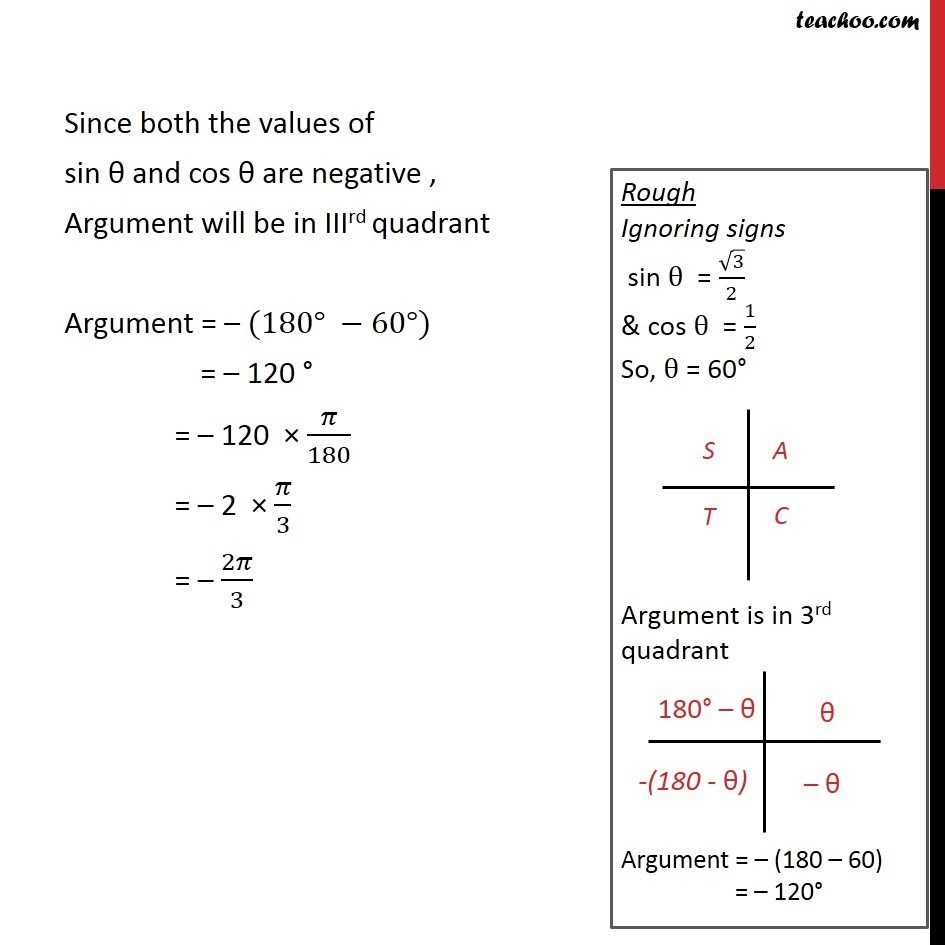1. Chapter 5 Class 11 Complex Numbers (Term 1)
2. Concept wise
3. Modulus,argument

Transcript

Ex 5.2, 1 Find the modulus and the argument of the complex number z = -1 - i 3 Method (1) for modulus z = 1 3 Complex number z is of the form x + y Here x = 1 , y = 3 Modulus of z = |z| = ( ^2+ ^2 ) = ("( 1)2 + ( " 3 ")2" ) = (1+3) = 4 = 2 Hence | | = 2 Modulus = 2 Method (2) for modulus z = 1 3 Let z = r (cos + sin ) Here r is modulus, and is argument From (1) & (2) 1 3 = r ( cos + sin ) 1 3 = r cos + r sin Comparing Real parts 1 = cos Squaring both sides ( -1 )2 = ( r cos )2 1 = r2 cos"2" Adding (3) & (4) 1 + 3 = 2 cos2 + r2 sin2 4 = 2 cos2 + r2 sin2 4 = 2 ( cos2 + sin2 ) 4 = 2 1 4 = 2 2 = 4 = 4 = 2 Hence, modulus = r = 2 Finding argument 1 3 = r cos + r sin Comparing real parts 1 = cos Putting r = 2 1 = 2 cos 1 = 2cos 1/2 = cos cos = 1/2 Hence, sin = 3/2 & cos = 1/2 Since both the values of sin and cos are negative , Argument will be in IIIrd quadrant Argument = (180 60 ) = 120 = 120 /180 = 2 /3 = 2 /3